Function Repository Resource:

# TileBins

Bin data into rectangular tiles

Contributed by: Anton Antonov
 ResourceFunction["TileBins"][mat,s] bins a numerical full 2D array mat into square grid tiles with size s. ResourceFunction["TileBins"][mat,{hx,hy}] bins a numerical full 2D array mat into rectangular grid tiles with x-y sizes {hx,hy}. ResourceFunction["TileBins"][cv,s] bins coordinates-to-value rules cv.

## Details and Options

ResourceFunction["TileBins"] bins data into rectangular tiles.
The result is an association with keys that are polygon objects.
ResourceFunction["TileBins"] accepts the following options:
 "AggregationFunction" Total function to aggregate the data in each tile "PolygonKeys" True determine whether the result keys are polygons
If the option "PolygonKeys" is set to False, then the keys of the result are rectangle centers.
The option "AggregationFunction" is used to aggregate the values within each tile only when coordinates-to-value rules are supplied.

## Examples

### Basic Examples (5)

Create a list of random 2D points:

 In:=Bin the points:

 In:=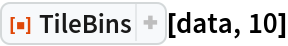Out=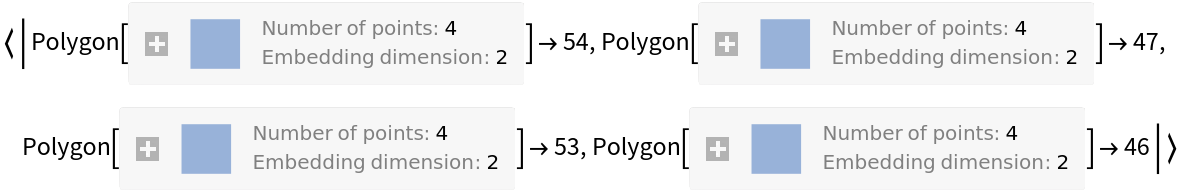Bin the points and get the tile centers as keys:

 In:=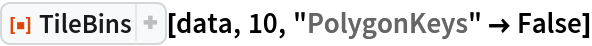Out=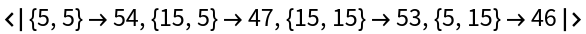Plot points and square bins:

 In:=Out=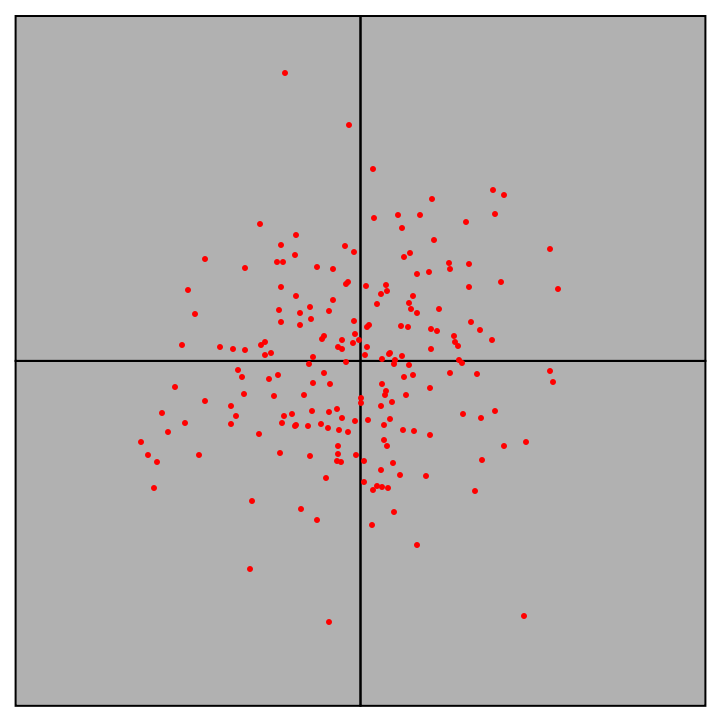Plot points and rectangle bins:

 In:=Out=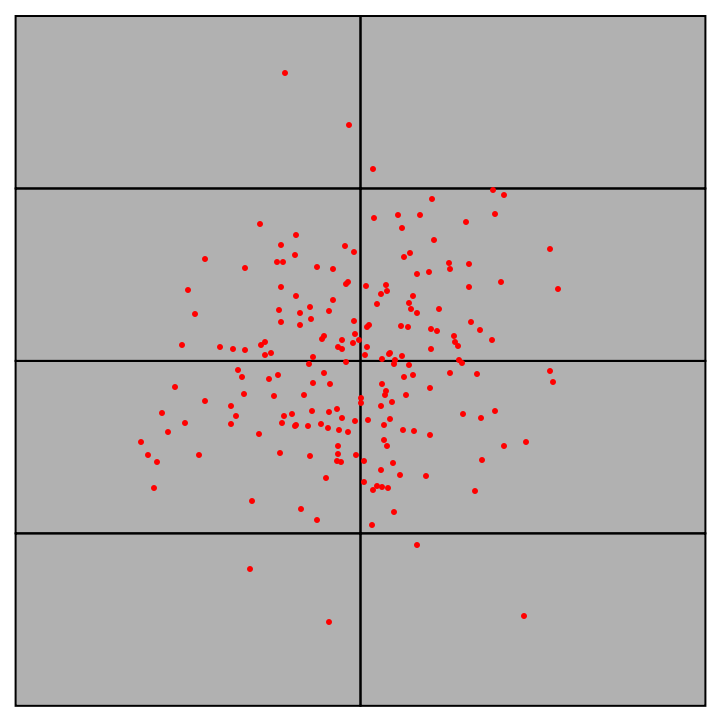### Scope (4)

Associate random values to 2D data:

 In:=Bin the data rules:

 In:=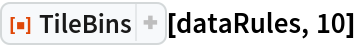Out=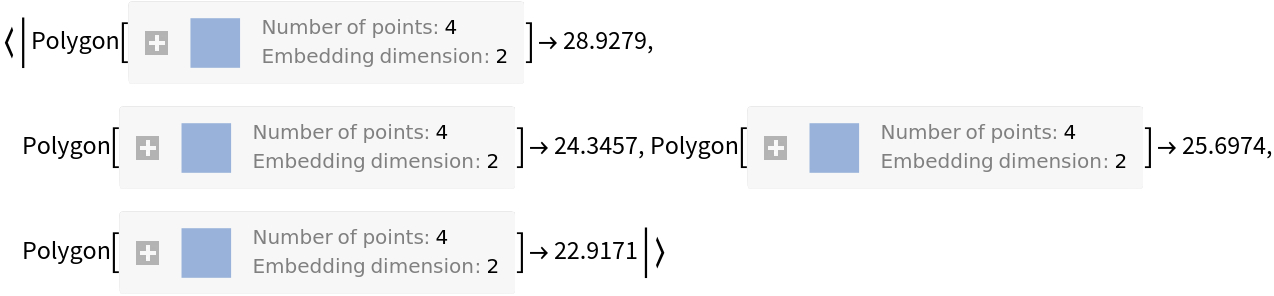Bin the keys of the data rules:

 In:=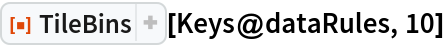Out=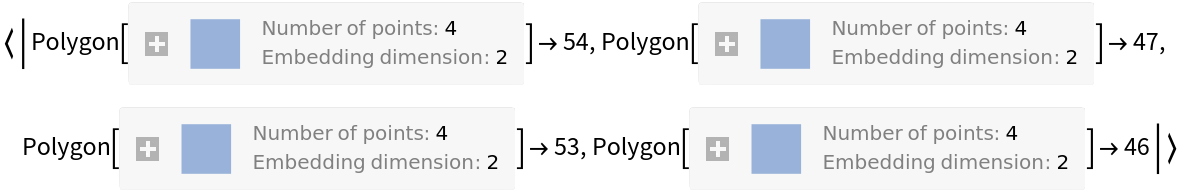Check the equivalence:

 In:=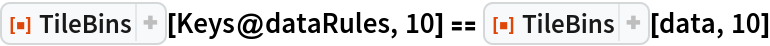Out=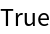### Options (3)

#### AggregationFunction (2)

Bin the data rules and compute average values on each tile:

 In:=Out=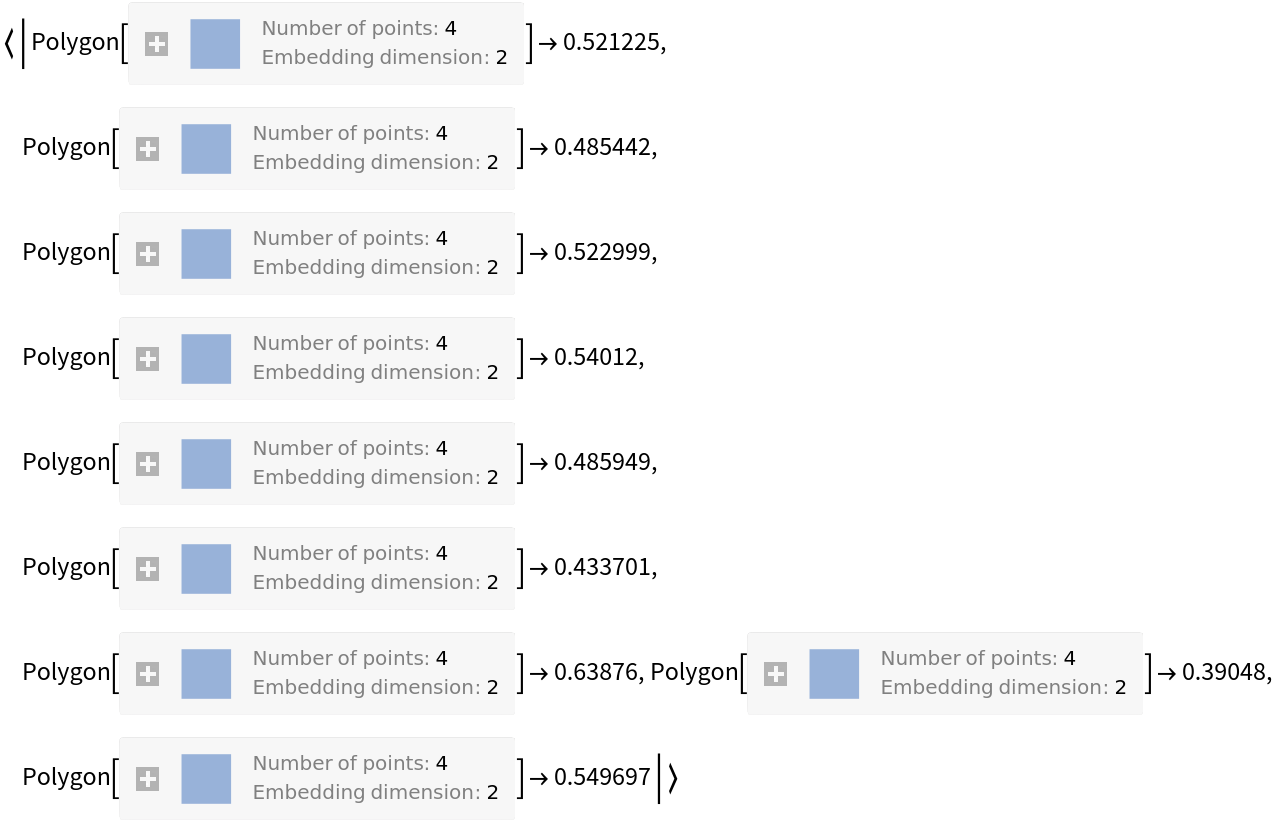Compare with the default option value, Total:

 In:=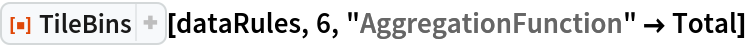Out=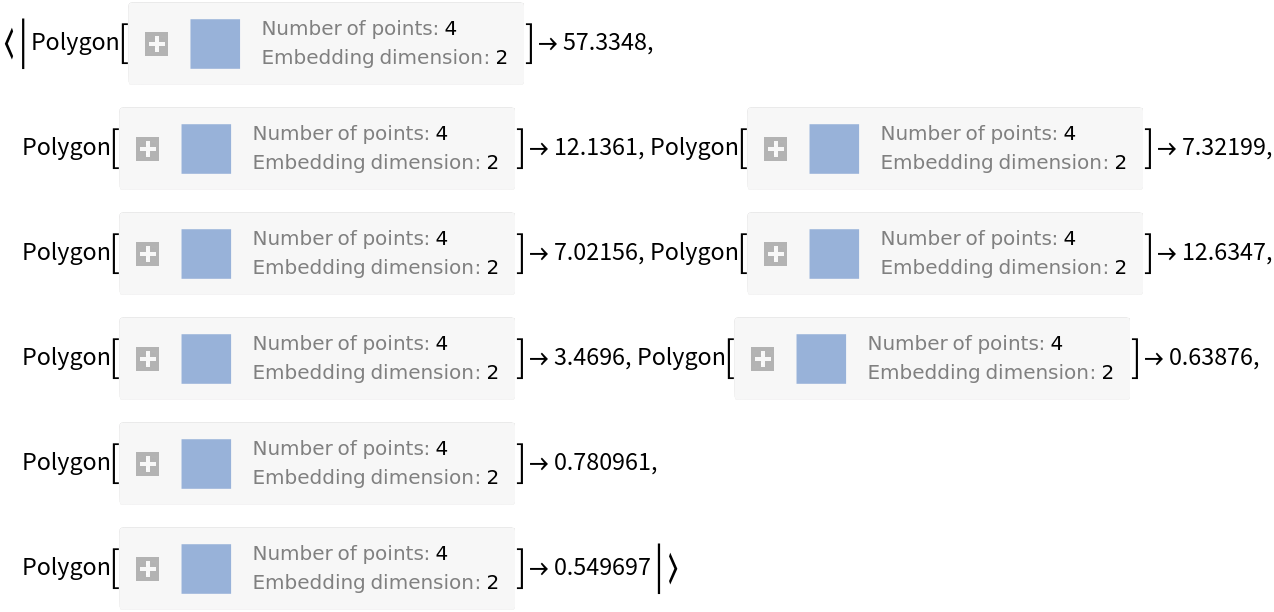#### PolygonKeys (1)

Instead of polygons as keys, you can get the centers of the polygons:

 In:=Out=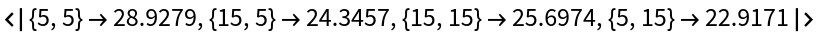### Applications (6)

#### Data visualization (4)

Generate data rules:

 In:=Here we bin the data:

 In:=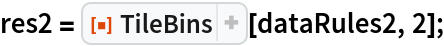Plot points and square bins by coloring each square according to its aggregated value:

 In:=Out=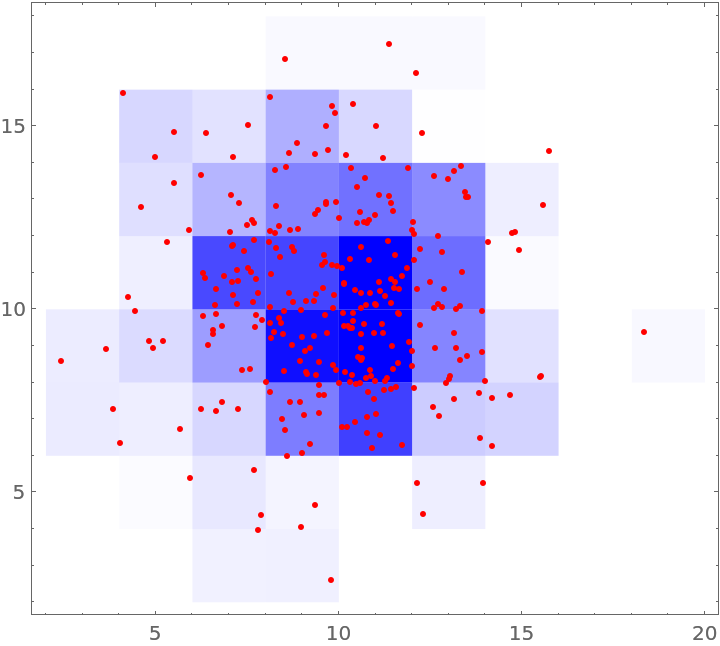Repeat the visualization above with rectangular tiles instead of square ones:

 In:=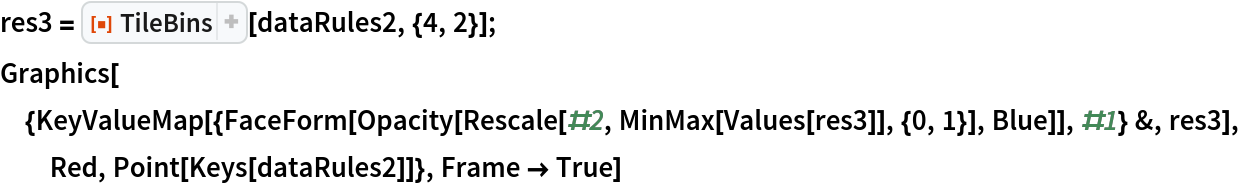Out=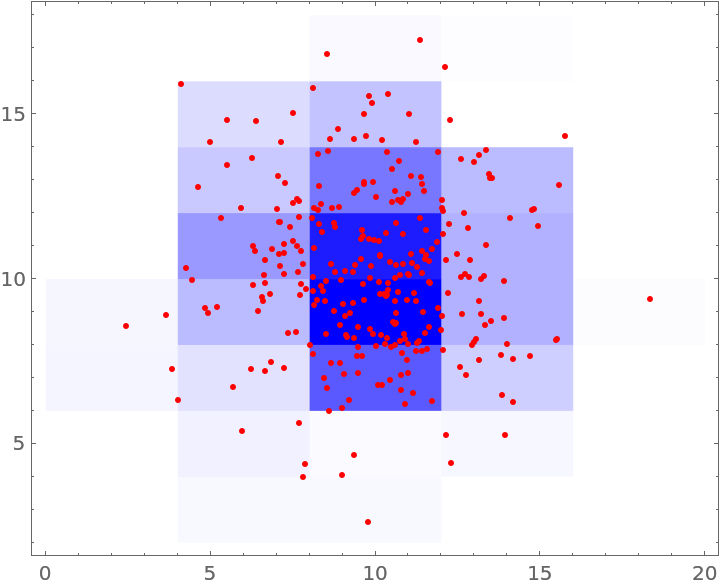#### Make a rectangular grid (2)

You can make rectangular grids:

 In:=Out=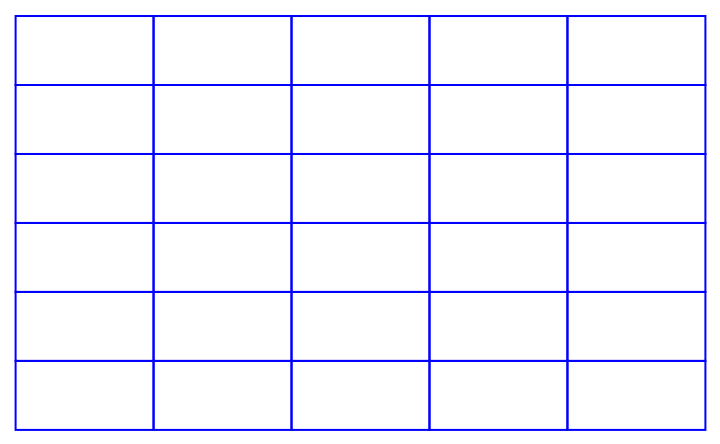The coordinates of the polygons can be retrieved and transformed further:

 In:=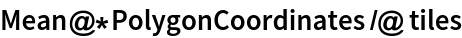Out=### Properties and Relations (3)

The repository function HextileBins has similar design and implementation as TileBins. Here are comparison examples:

 In:=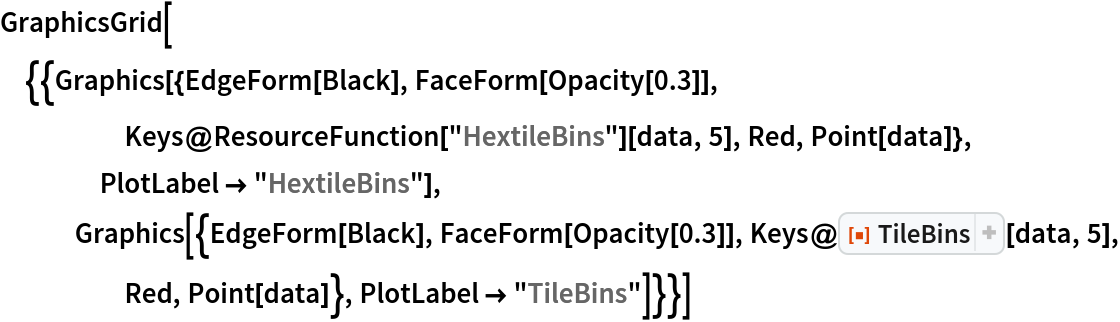Out=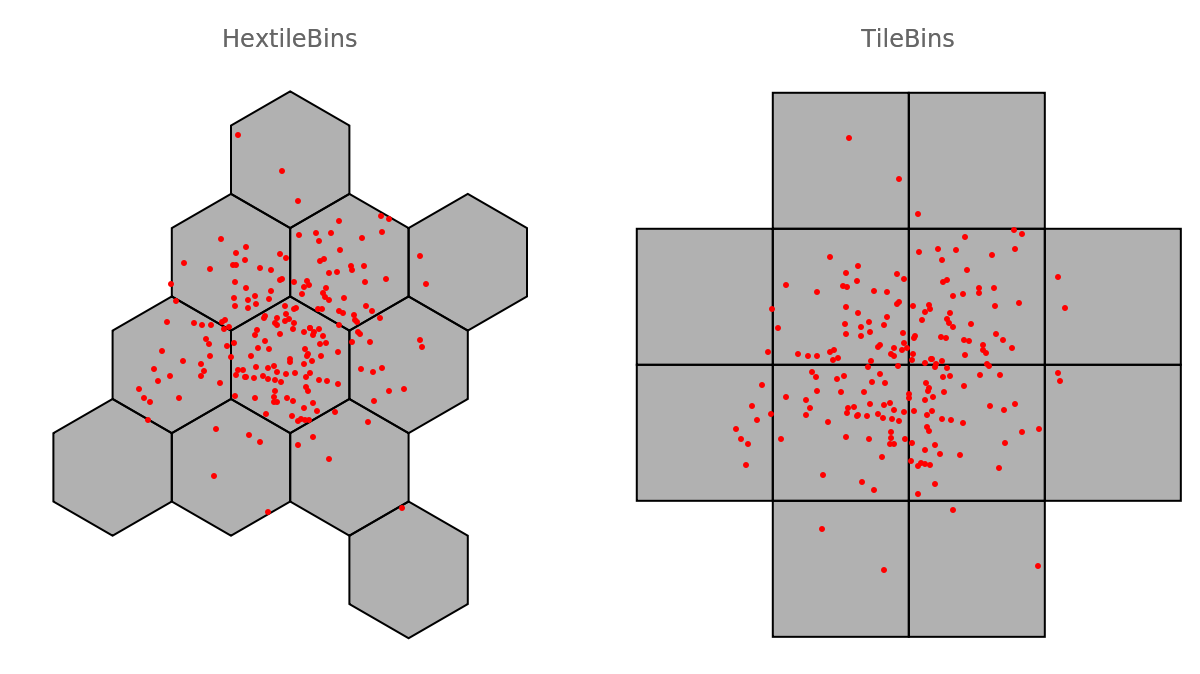The function GeoHistogram produces similar plots (in Version 12.1):

 In:=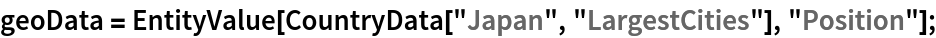In:=In:=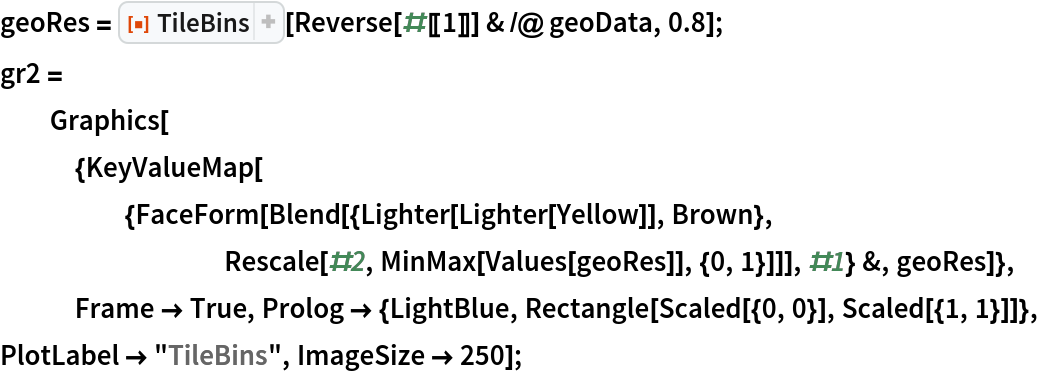In:=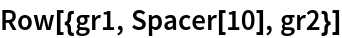Out=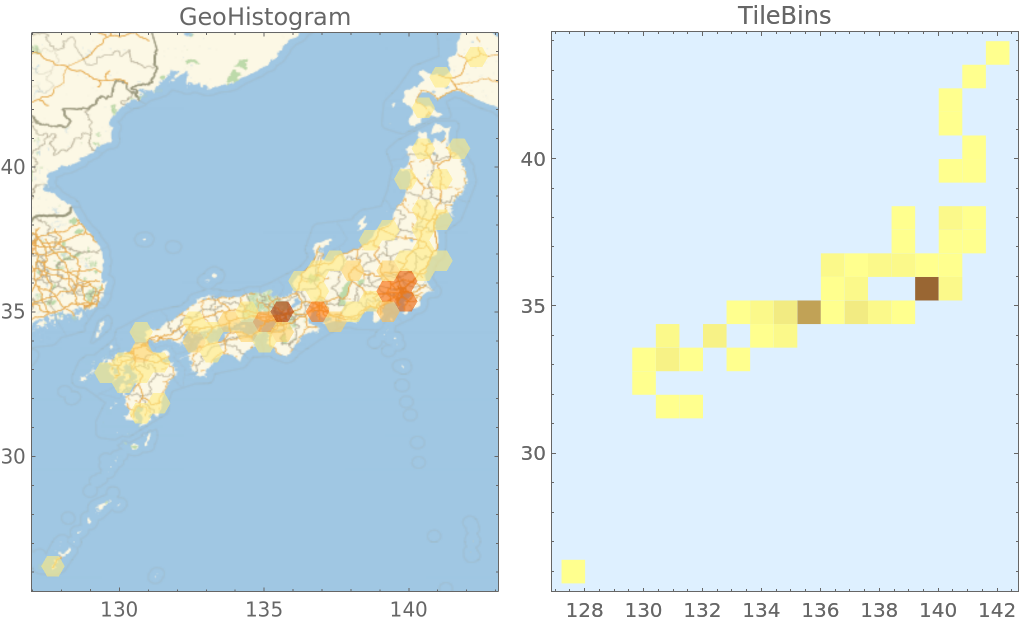The function GridGraph can be also used to make rectangular grids:

 In:=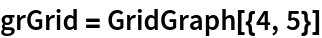Out=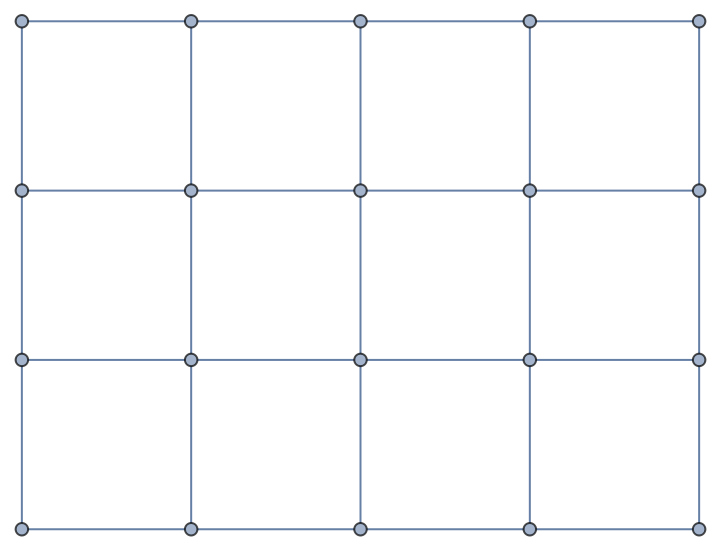Here are the corresponding coordinates:

 In:=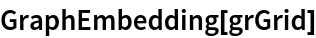Out=Here are the corresponding polygons:

 In:=Out=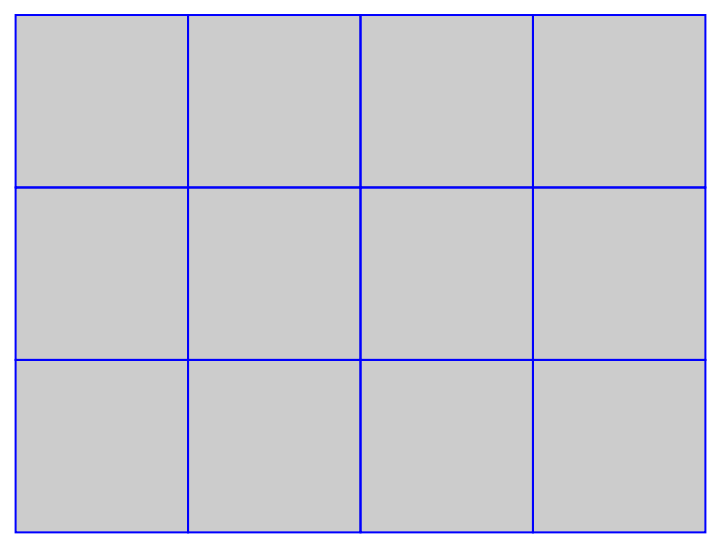Make a similar grid with TileBins:

 In:=Out=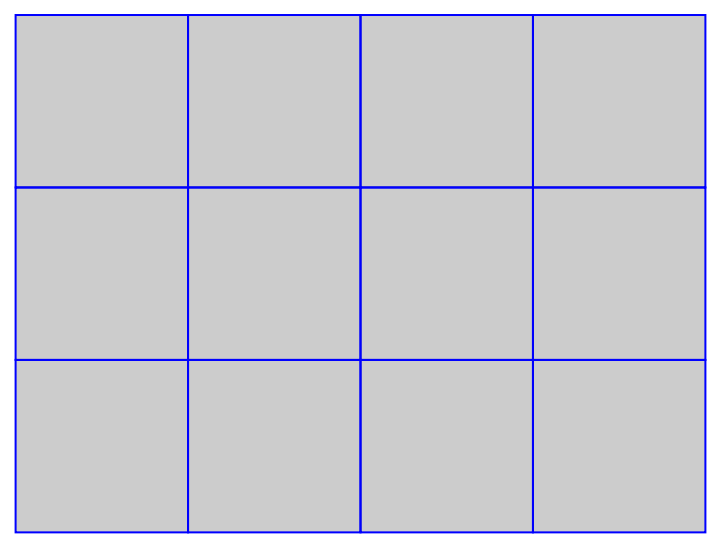Here are the corresponding coordinates:

 In:=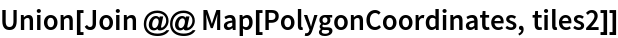Out=### Neat Examples (1)

Bin data and summarize the values associated to bin centers:

 In:=In:=Out=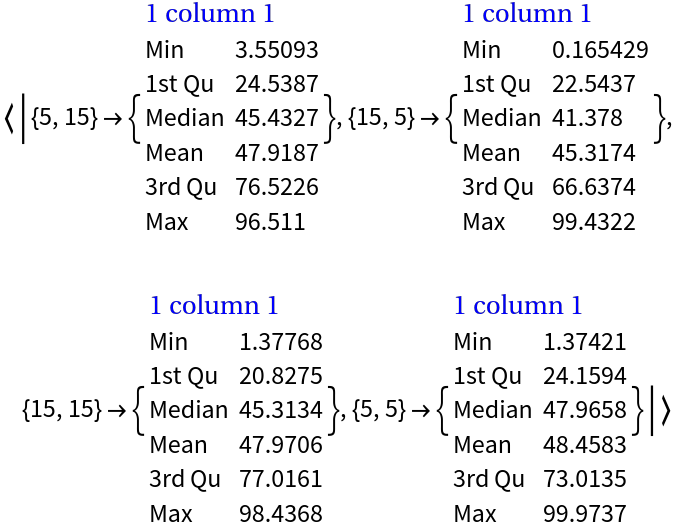Anton Antonov

## Version History

• 1.0.0 – 05 May 2020